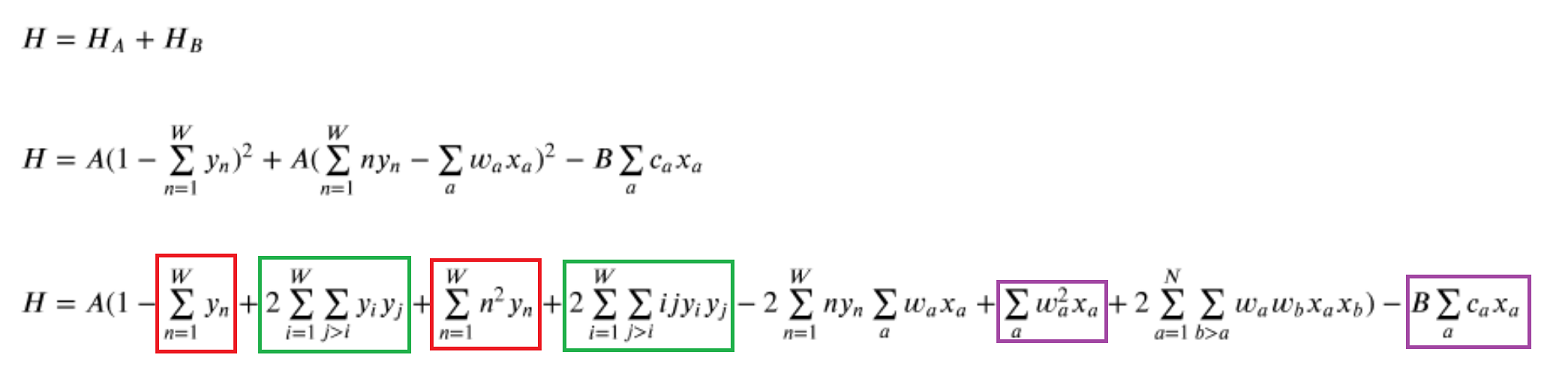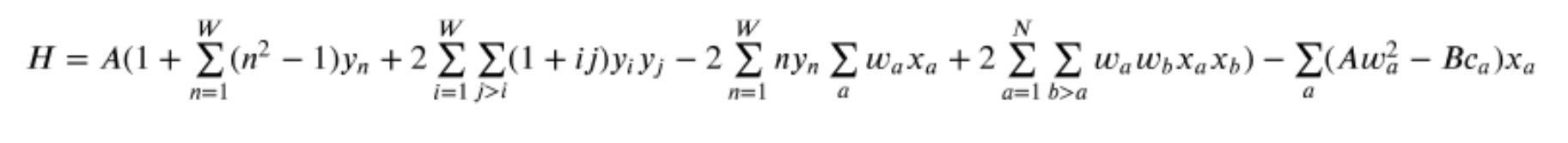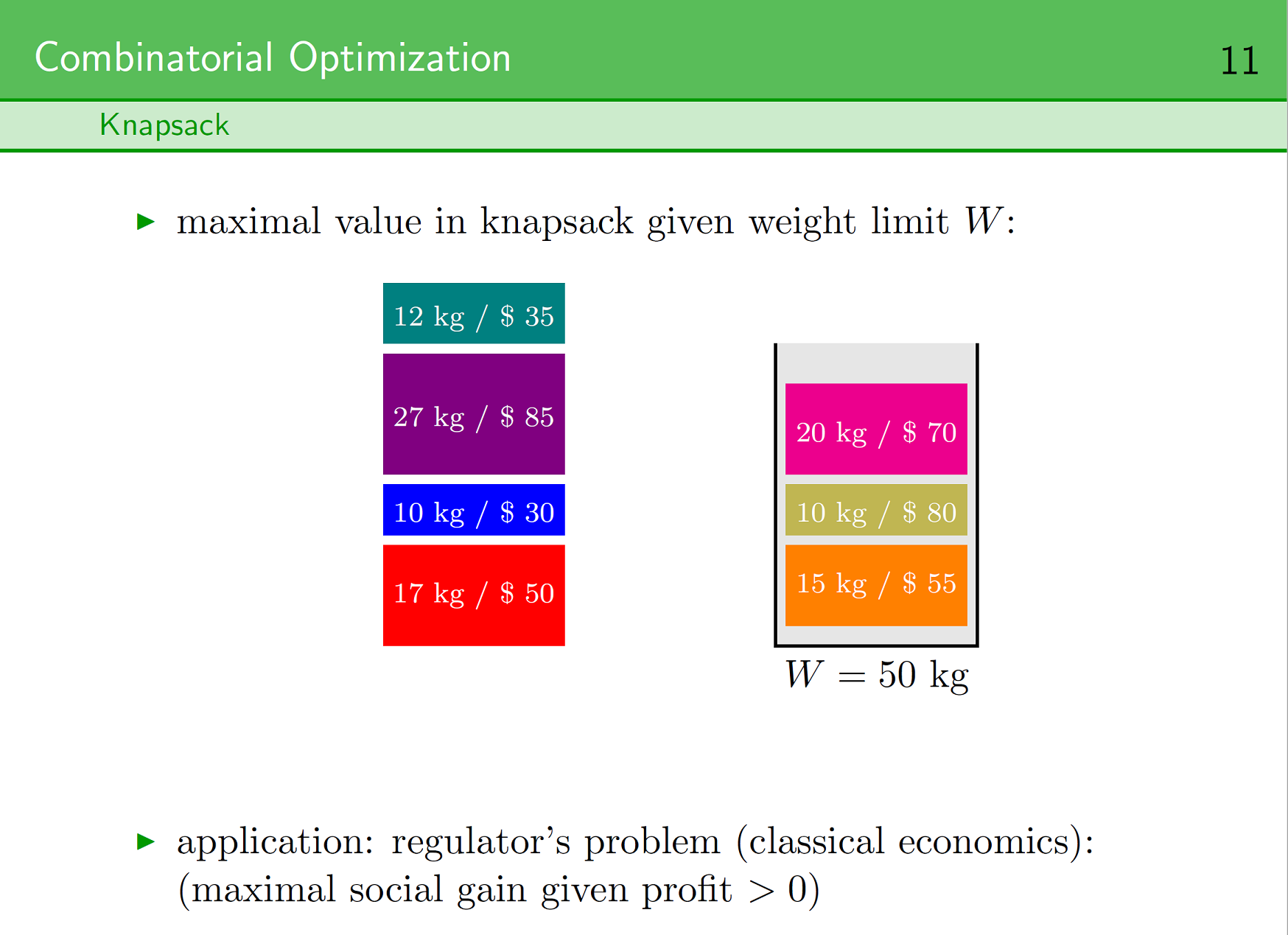# Knapsack problem

I've tried to solve the Knapsack problem the other day but without the so called "trick" from Andrew Lucas (https://www.frontiersin.org/articles/10.3389/fphy.2014.00005/full, 2.4. Reducing N to logN Spins in Some Constraints, 5.2. Knapsack with Integer Weights).

I could not solve it so far I'm still trying and just wondering whether it can be solved or not without the "trick"? It should be possible, right?

From Lucas's paper I've converted the formulas and marked the constraints with letters so you can identify them in my approach:

My approach:

I've commented out the original solution and added my constraints next to the original constraints so you can compare them. They look similar except I don't use the "trick" and 2 of my constraints (a and b) are missing from the original solution.

Is my approach correct at all? Any suggestions?

1 comment
• Hi Imre,

It took me a little while to figure out what was happening with your code! Your math is correct and it is possible to implement Lucas' solution without the 'trick'. The issue you're seeing is that your code doesn't reflect the QUBO equation. Notice that in the equation below (the same equation you found) there are duplicate terms, or terms that can be combined and simplified (these are highlighted in the colored boxes).When you enter each of the 8 terms into your QUBO dictionary separately (as you did in your code), you end up overwriting some of your terms. For example, if you add the entries from the first red box in a 'for' loop first and then you add the terms from the second red box in a separate 'for' loop, you overwrite the terms from the first 'for' loop.

`# Terms from the first red box (labelled 'a' in your equation)for n in range(weight_capacity):    bqm.set_linear('y' + str(n), lagrange * -1)# Terms from the second red box (labelled 'c' in your equation)# *** This overwrites the entries from above ***for n in range(weight_capacity):     bqm.set_linear('y' + str(n), lagrange * y[n] ** 2)`

Instead, you can simplify the equation, or group terms, like this:That way you're only adding entries to the dictionary once and you eliminate the risk of overwriting previous entries.

It may also be worth mentioning that D-Wave's knapsack example uses a slightly different implementation than you found in Andrew Lucas' paper. That implementation comes from a modification that Andrew Lucas spoke about in a presentation after he published his paper. Here are the relevant slides:I hope this helps!